# Question & Answer: Q3: For the network below to demonstrate forward propagation the inputs are (1, 1) & the target is (0) & apply the activation function (sigmoid function)f(Cx)to the hidden lay…..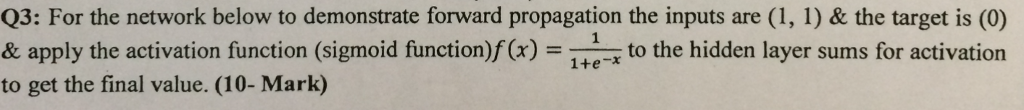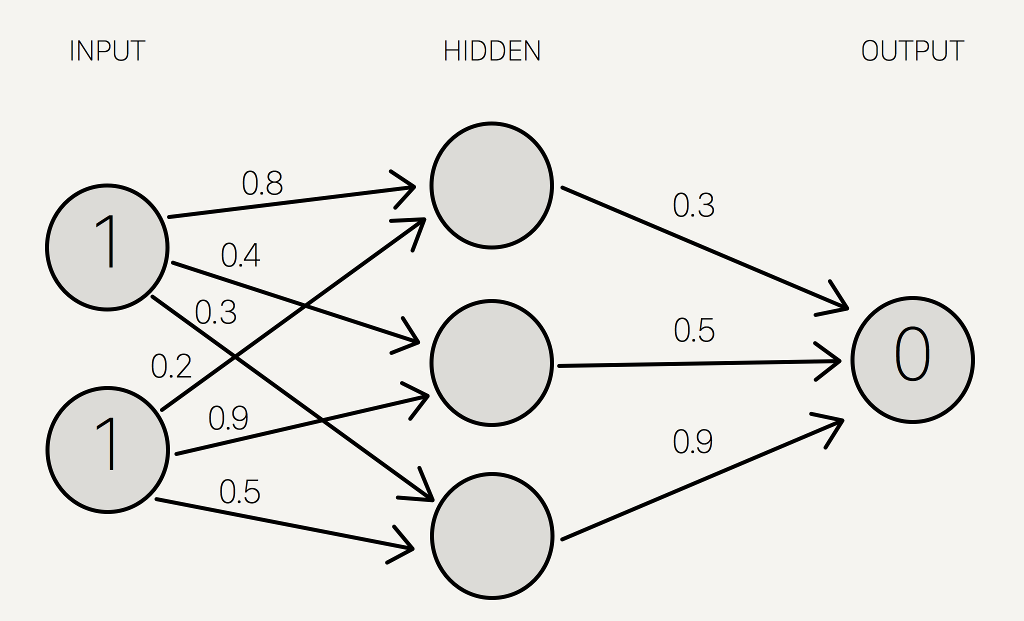Neural Network

Q3: For the network below to demonstrate forward propagation the inputs are (1, 1) & the target is (0) & apply the activation function (sigmoid function)f(Cx)to the hidden layer sums for activation to get the final value. (10- Mark)

Don't use plagiarized sources. Get Your Custom Essay on
Question & Answer: Q3: For the network below to demonstrate forward propagation the inputs are (1, 1) & the target is (0) & apply the activation function (sigmoid function)f(Cx)to the hidden lay…..
GET AN ESSAY WRITTEN FOR YOU FROM AS LOW AS \$13/PAGE

In Forward Propagation, we apply set of weights to the input data andcalculate an output.

Neutral Network repeats for both Forward and Backward Propagation until the desired output comes.

We use the X-OR ( Exclusive OR) Operation for solving the problem.
It would provide correct output given any input acceptable by XOR function. The table is as follows:

 Inputs Outputs 0, 0 0 0, 1 1 1, 0 1 1, 1 0

Let’s use last row from the table ( 1 , 1) => 0 for forward propagation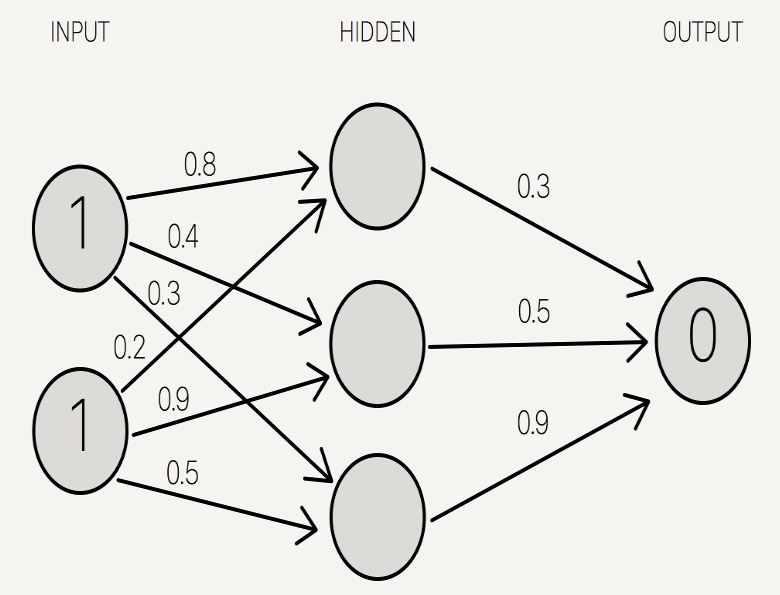We Sum the Product of inputs with their corresponding set opf weights arrive at the hidden layer as follows:

( 1 * 0.8 ) + ( 1 * 0.2 ) = 1

( 1 * 0.4 ) + ( 1 * 0.9 ) = 1.3

( 1 * 0.3 ) + ( 1 * 0.5 ) = 0.8

We put these sums in the circles of hidden layer as follows:-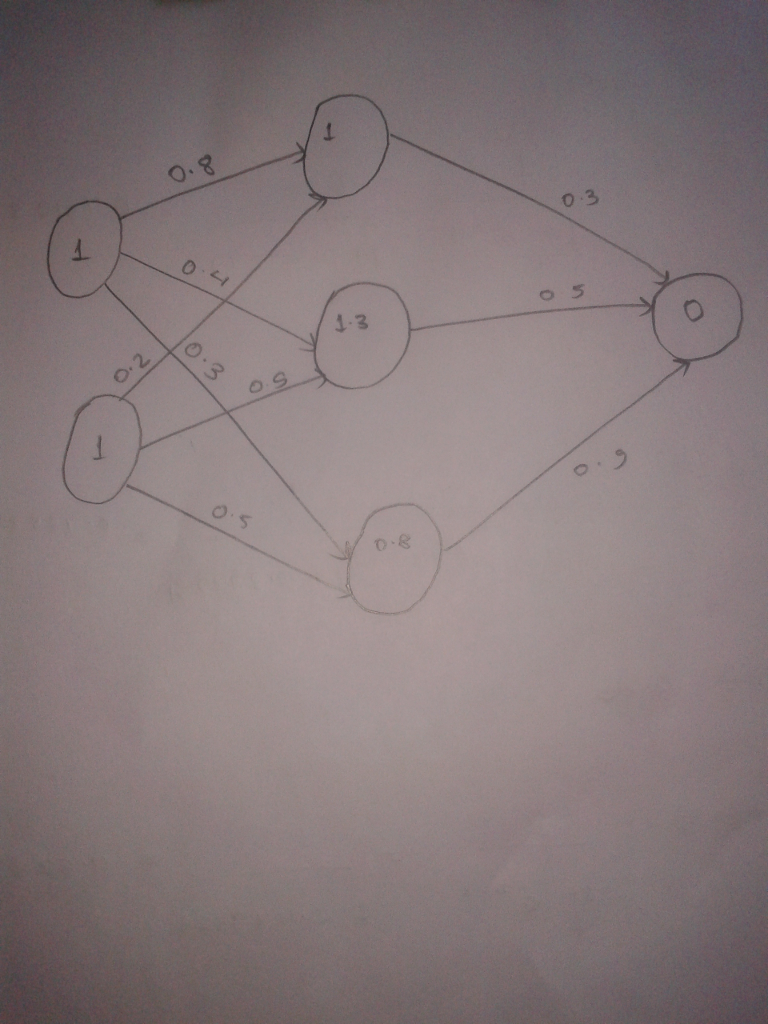Now to get the final value we apply the activation function i.e. sigmoid function to hidden layers sums. it’s purpose is to translate input signal to output soignals.

Sigmod Function : 1 / 1 + e-x

The 2D graphical representation of such function is as follows: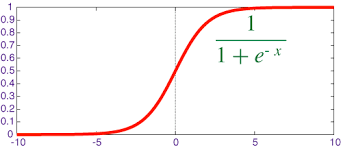Now applying Sigmod Function to the three hidden layers as follows: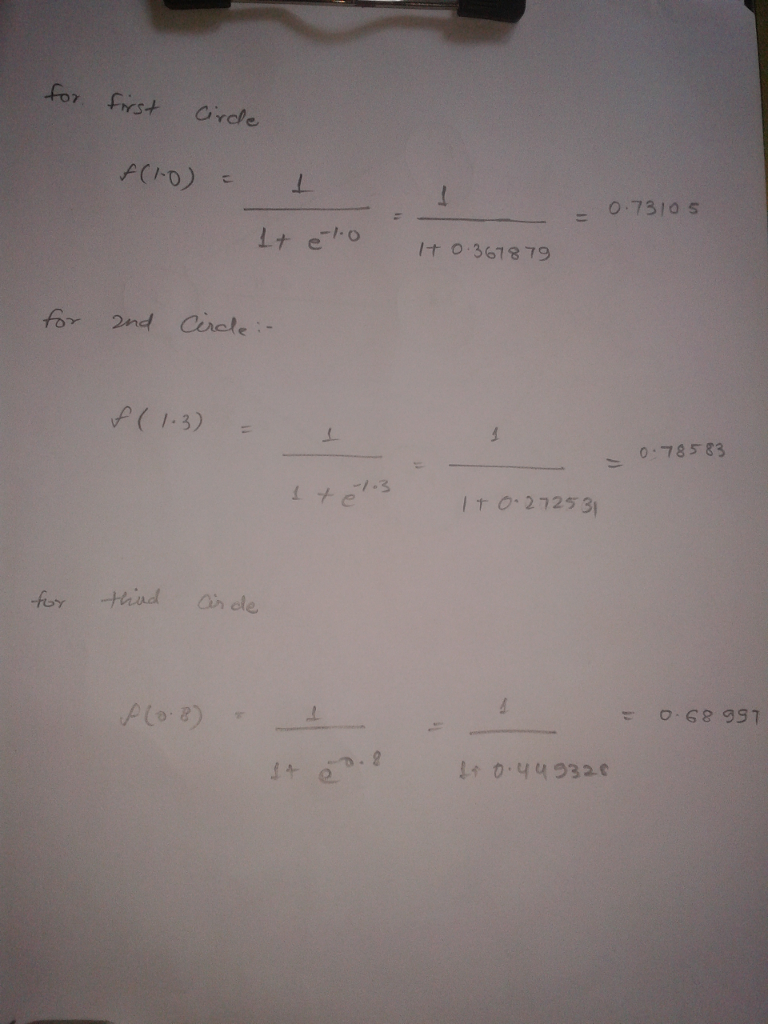Now we add these calculations to neutral network as hidden layer result: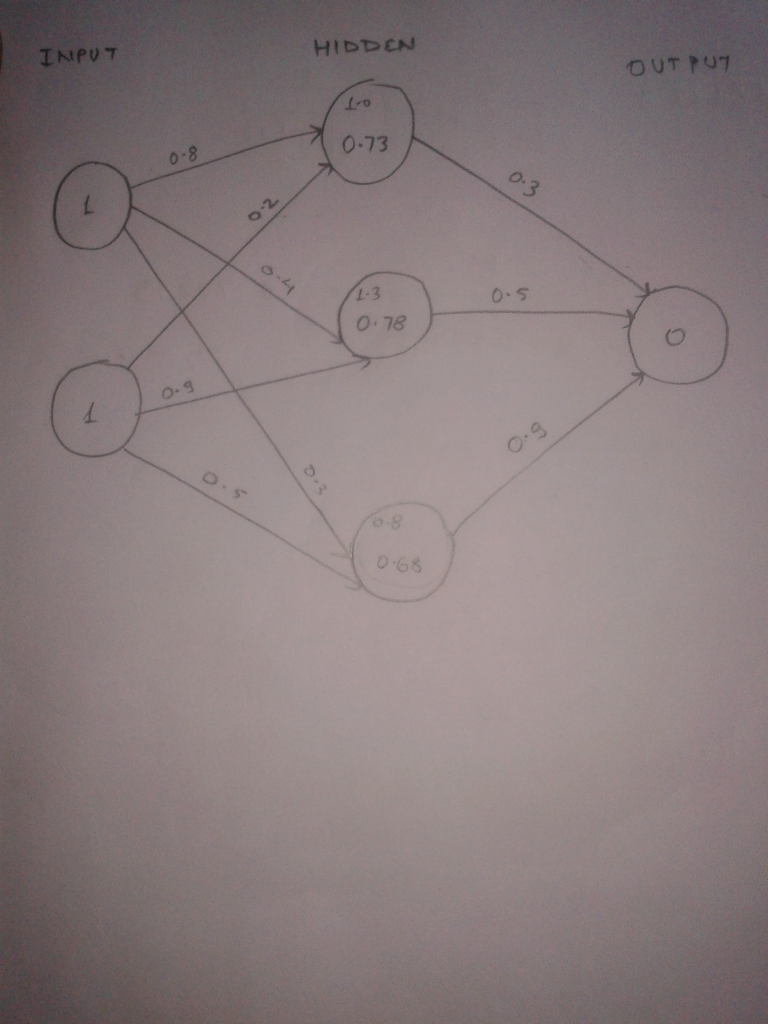Now we Calculate the SOP of hidden layers with second set of weights drom hidden to output layer as follows:

( 0.73 * 0.3 ) + ( 0.78 * 0.5) + ( 0.68 * 0.9 ) = 1.221

Now we finally calculate final output result by applying Sigmoid function as follows: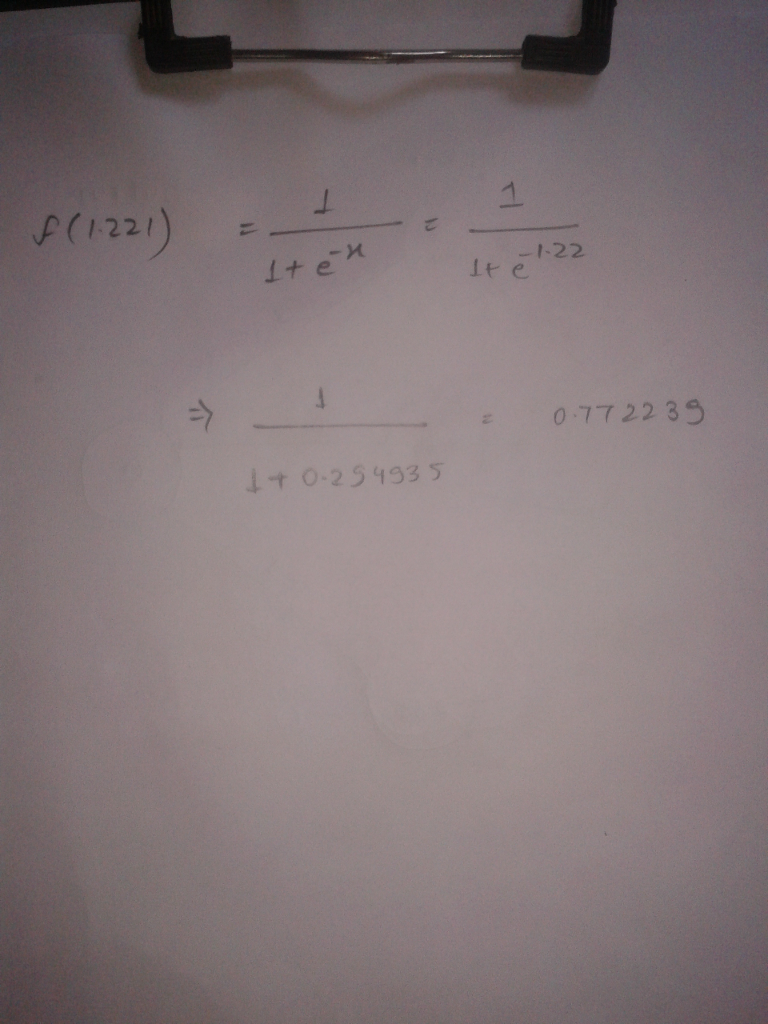The full diagram is as follows: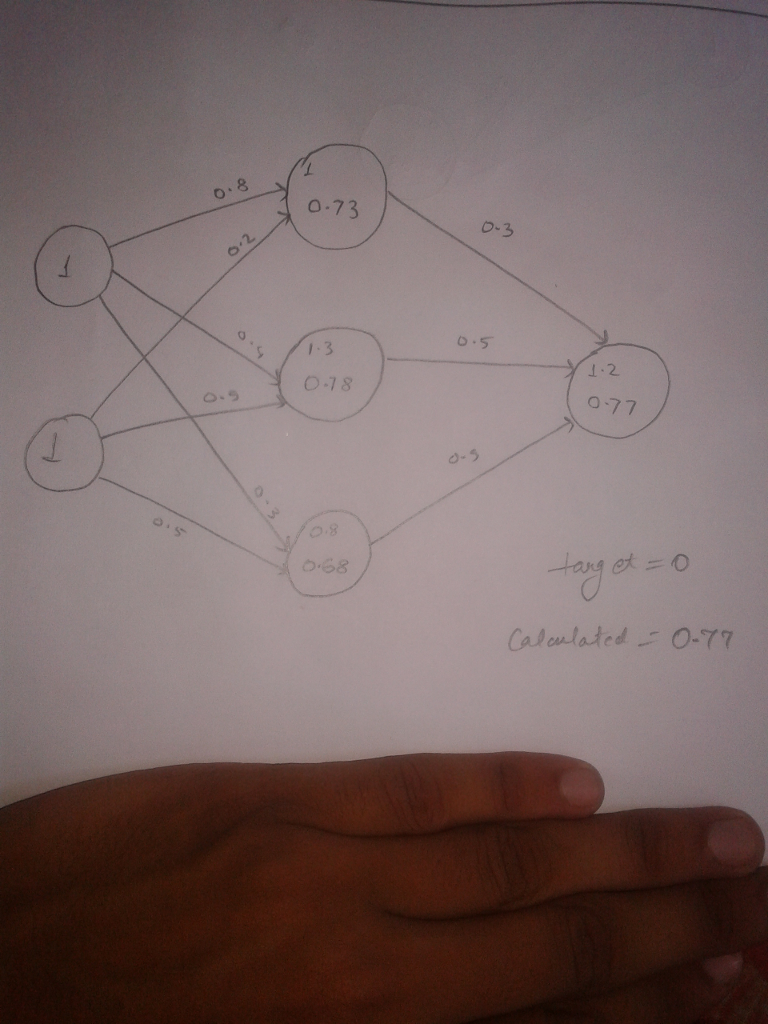We use the random set of initial weights so the output is off the mark in this case by +0.77. To get more accurate result you should use the Backward propagation. it doesn’t provide accurate result but sometimes near to the solution.